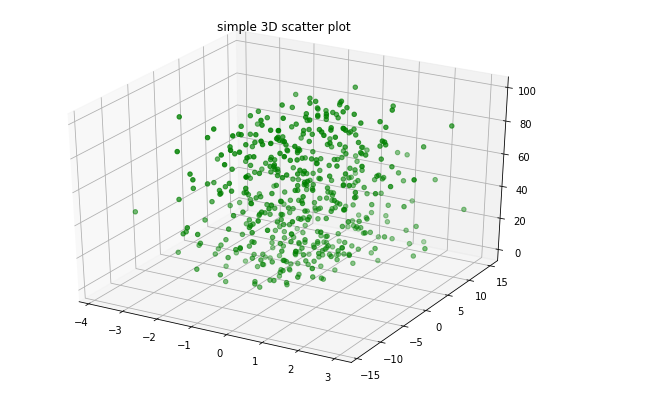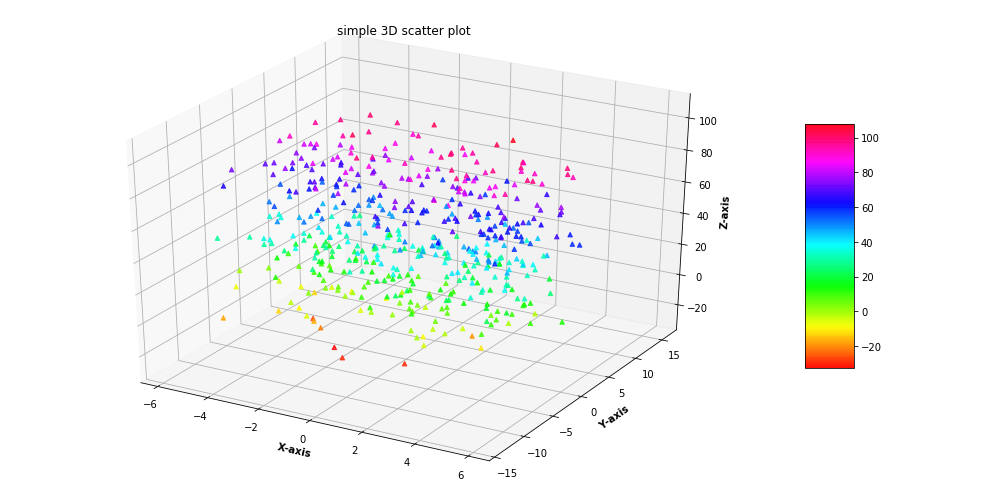# 3D Scatter Plotting in Python using Matplotlib

A 3D Scatter Plot is a mathematical diagram, the most basic version of three-dimensional plotting used to display the properties of data as three variables of a dataset using the cartesian coordinates.To create a 3D Scatter plot, Matplotlib’s mplot3d toolkit is used to enable three dimensional plotting.Generally 3D scatter plot is created by using `ax.scatter3D()` the function of the matplotlib library which accepts a data sets of X, Y and Z to create the plot while the rest of the attributes of the function are the same as that of two dimensional scatter plot.

Example 1: Let’s create a basic 3D scatter plot using the `ax.scatter3D()` function.

 `# Import libraries ` `from` `mpl_toolkits ``import` `mplot3d ` `import` `numpy as np ` `import` `matplotlib.pyplot as plt ` ` `  ` `  `# Creating dataset ` `z ``=` `np.random.randint(``100``, size ``=``(``50``)) ` `x ``=` `np.random.randint(``80``, size ``=``(``50``)) ` `y ``=` `np.random.randint(``60``, size ``=``(``50``)) ` ` `  `# Creating figure ` `fig ``=` `plt.figure(figsize ``=` `(``10``, ``7``)) ` `ax ``=` `plt.axes(projection ``=``"3d"``) ` ` `  `# Creating plot ` `ax.scatter3D(x, y, z, color ``=` `"green"``); ` `plt.title(``"simple 3D scatter plot"``) ` ` `  `# show plot ` `plt.show() `

Output :Example 2 : For better understanding Let’s take another example.

 `# Import libraries ` `from` `mpl_toolkits ``import` `mplot3d ` `import` `numpy as np ` `import` `matplotlib.pyplot as plt ` ` `  ` `  `# Creating dataset ` `z ``=` `4` `*` `np.tan(np.random.randint(``10``, size ``=``(``500``))) ``+` `np.random.randint(``100``, size ``=``(``500``)) ` `x ``=` `4` `*` `np.cos(z) ``+` `np.random.normal(size ``=` `500``) ` `y ``=` `4` `*` `np.sin(z) ``+` `4` `*` `np.random.normal(size ``=` `500``) ` ` `  `# Creating figure ` `fig ``=` `plt.figure(figsize ``=` `(``16``, ``9``)) ` `ax ``=` `plt.axes(projection ``=``"3d"``) ` `   `  `# Add x, y gridlines  ` `ax.grid(b ``=` `True``, color ``=``'grey'``,  ` `        ``linestyle ``=``'-.'``, linewidth ``=` `0.3``,  ` `        ``alpha ``=` `0.2``)  ` ` `  ` `  `# Creating color map ` `my_cmap ``=` `plt.get_cmap(``'hsv'``) ` ` `  `# Creating plot ` `sctt ``=` `ax.scatter3D(x, y, z, ` `                    ``alpha ``=` `0.8``, ` `                    ``c ``=` `(x ``+` `y ``+` `z),  ` `                    ``cmap ``=` `my_cmap,  ` `                    ``marker ``=``'^'``) ` ` `  `plt.title(``"simple 3D scatter plot"``) ` `ax.set_xlabel(``'X-axis'``, fontweight ``=``'bold'``)  ` `ax.set_ylabel(``'Y-axis'``, fontweight ``=``'bold'``)  ` `ax.set_zlabel(``'Z-axis'``, fontweight ``=``'bold'``) ` `fig.colorbar(sctt, ax ``=` `ax, shrink ``=` `0.5``, aspect ``=` `5``) ` ` `  `# show plot ` `plt.show() `

Output :My Personal Notes arrow_drop_upCheck out this Author's contributed articles.

If you like GeeksforGeeks and would like to contribute, you can also write an article using contribute.geeksforgeeks.org or mail your article to contribute@geeksforgeeks.org. See your article appearing on the GeeksforGeeks main page and help other Geeks.

Please Improve this article if you find anything incorrect by clicking on the "Improve Article" button below.

Article Tags :

Be the First to upvote.

Please write to us at contribute@geeksforgeeks.org to report any issue with the above content.